# RD Sharma Solutions For Class 7 Maths Chapter 23 Data Handling - II (Central Values) Exercise 23.2

Students can view and download the PDF of RD Sharma Solutions for Class 7 Maths Exercise 23.2 of Chapter 23 Data Handling – II available here. The BYJU’S experts in Maths formulate the solutions for questions present in the textbook based on the understanding abilities of students. Exercise 23.2 mainly explains about the exterior and interior angles of a triangle. RD Sharma Solutions for Class 7 PDF can be downloaded by the students and referred to while solving the textbook problems. This exercise also includes the arithmetic mean of grouped data.

## Download the PDF of RD Sharma Solutions For Class 7 Maths Chapter 23 – Data Handling – II (Central Values) Exercise 23.2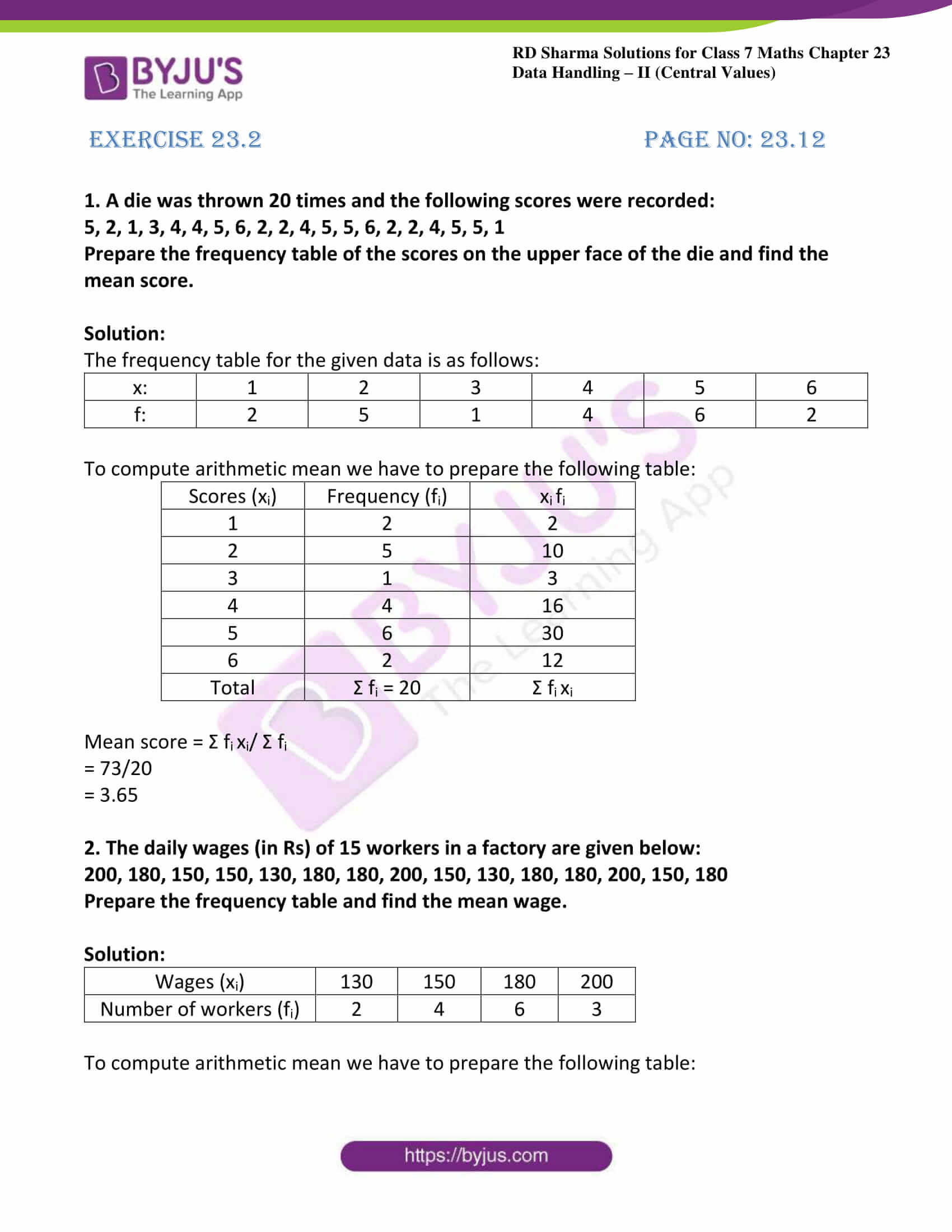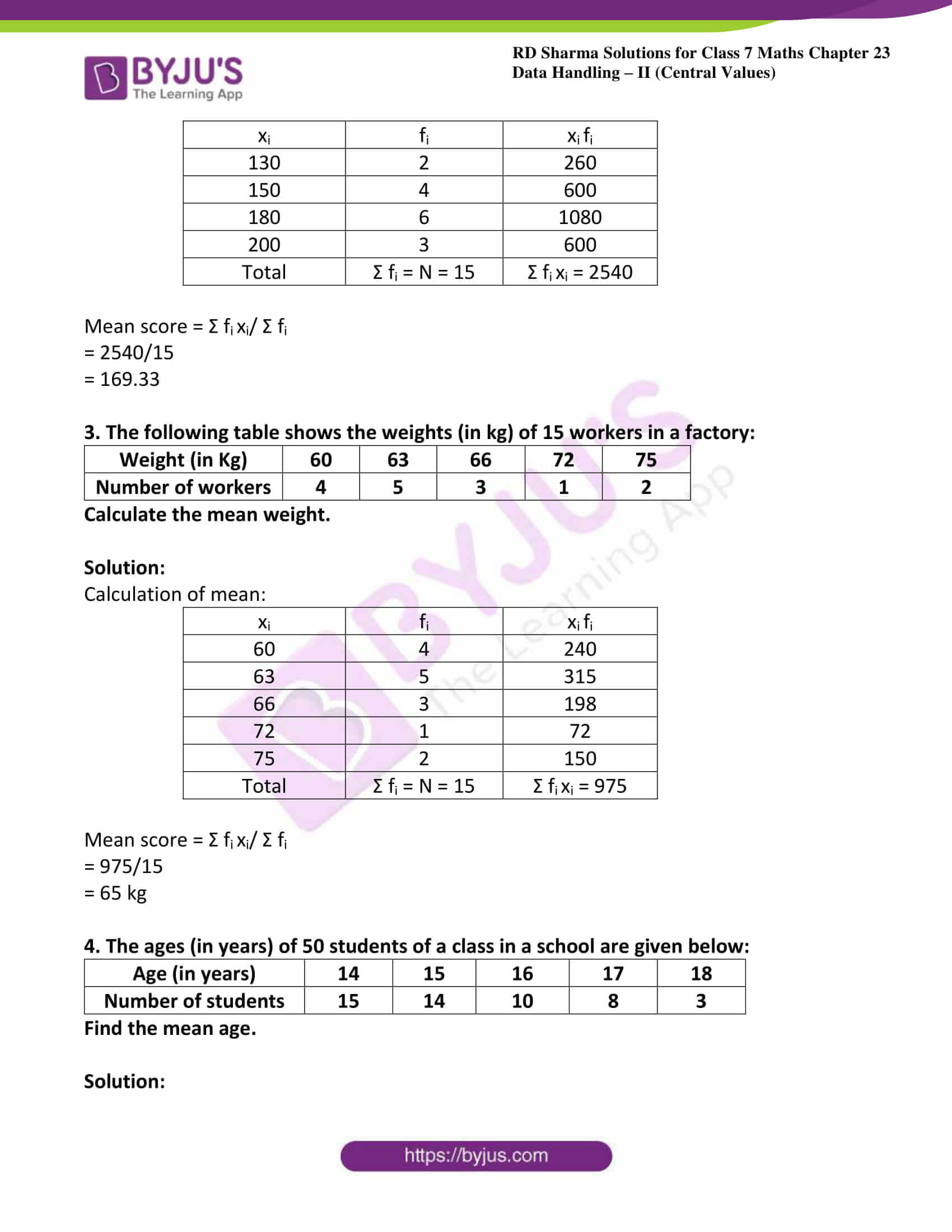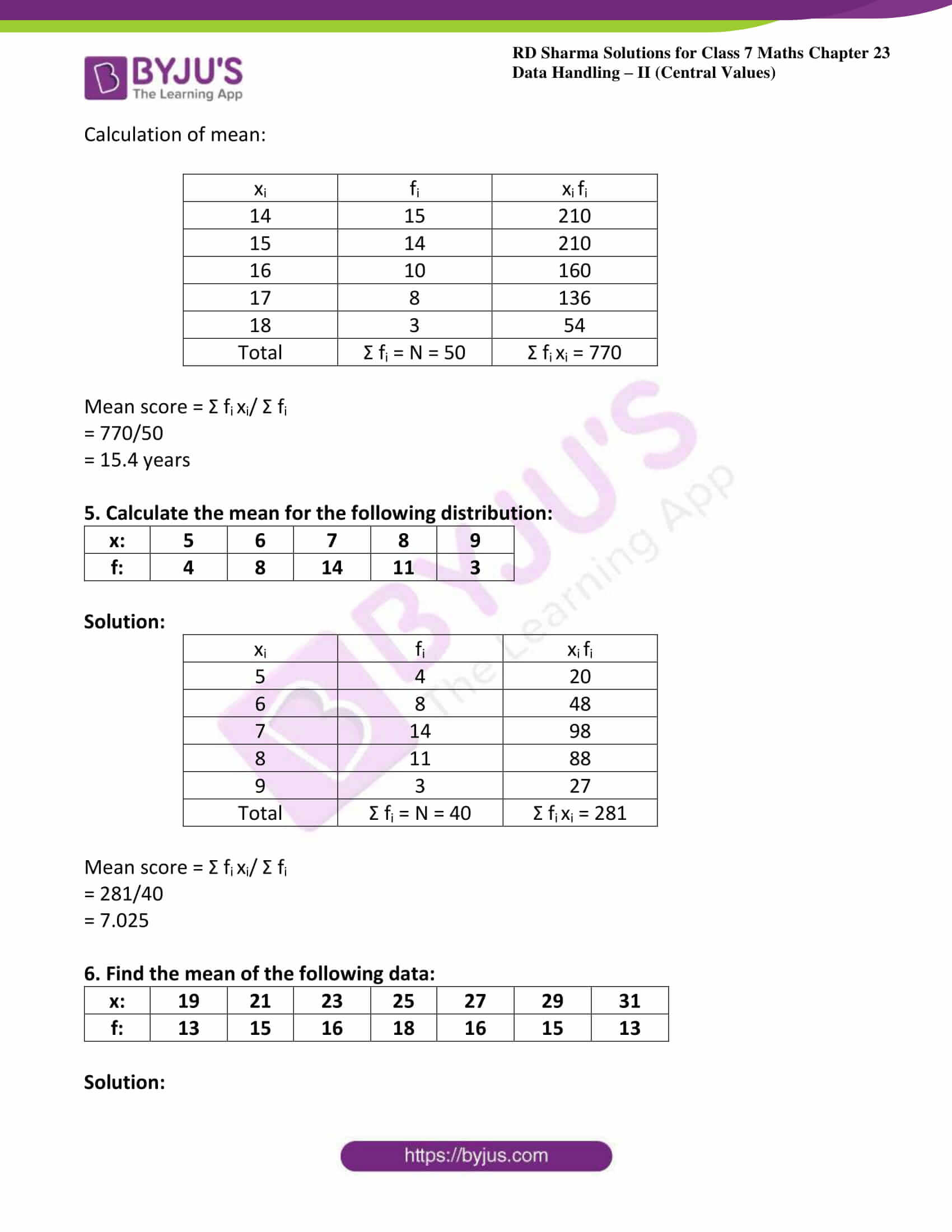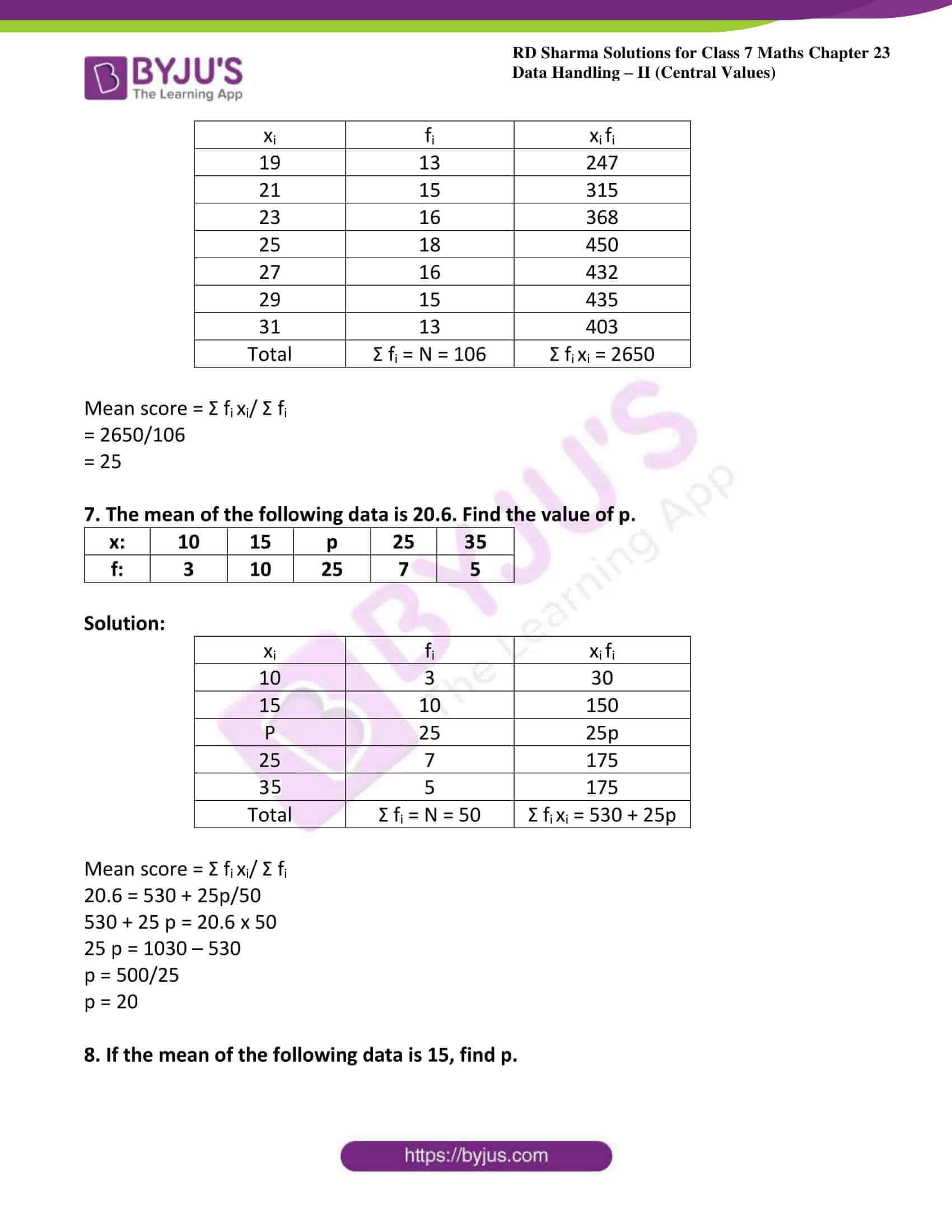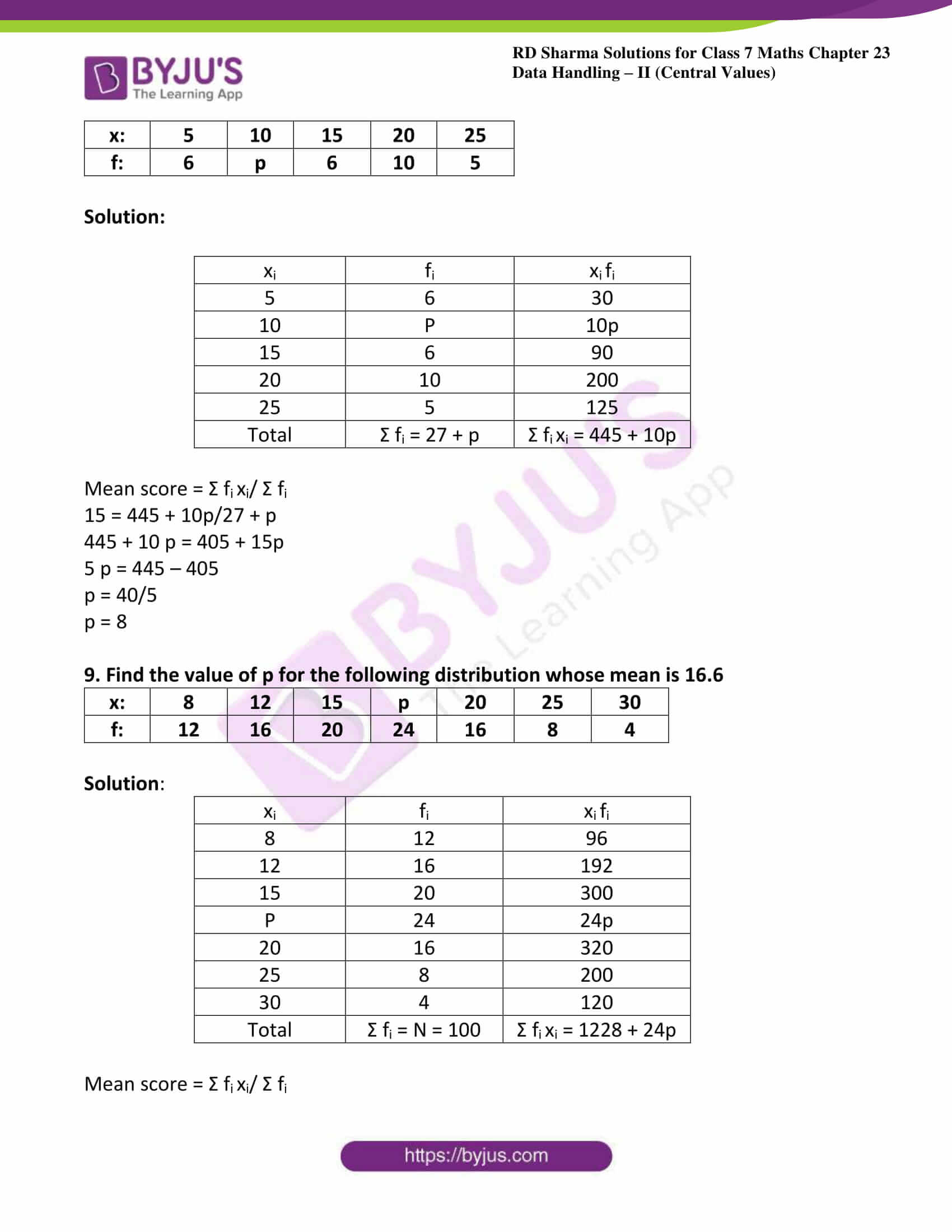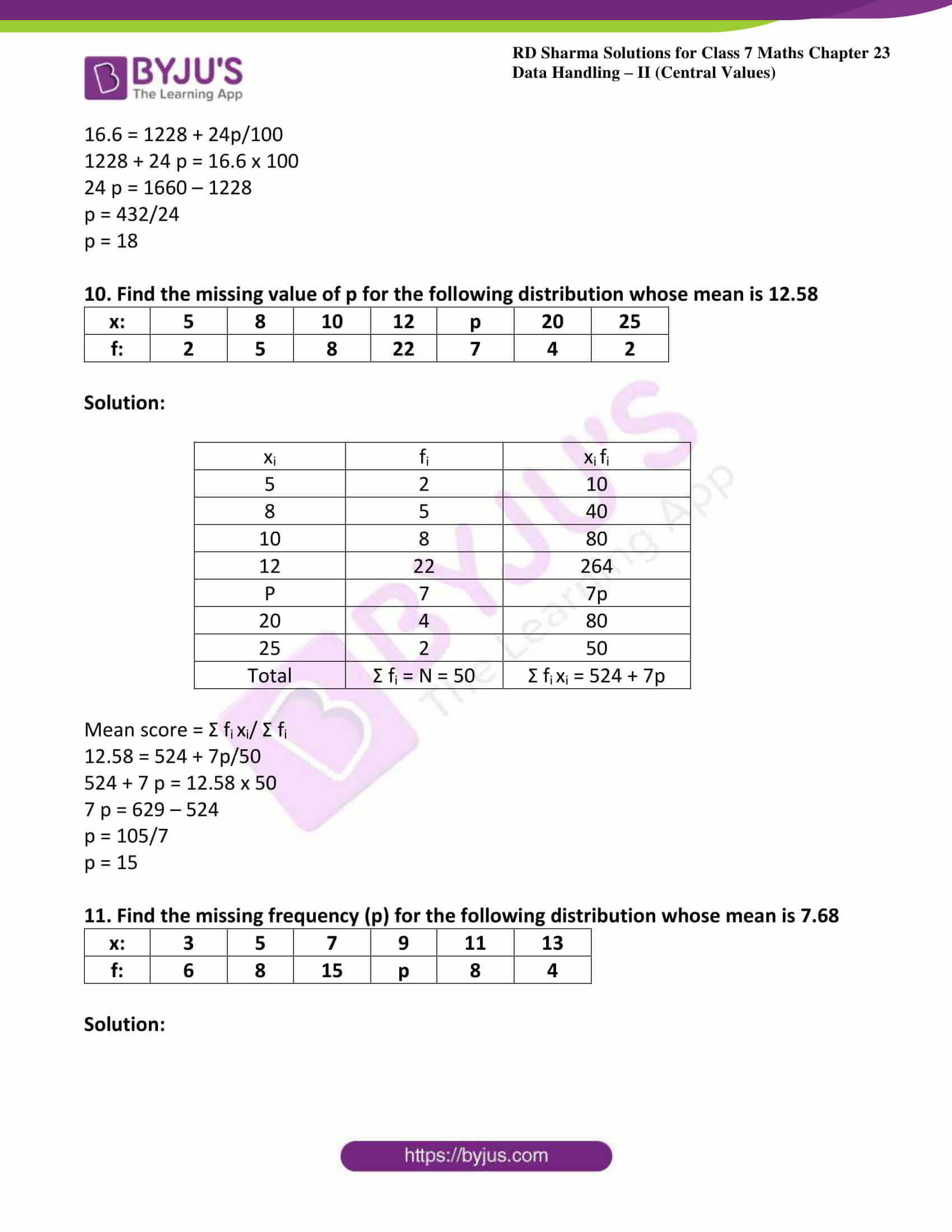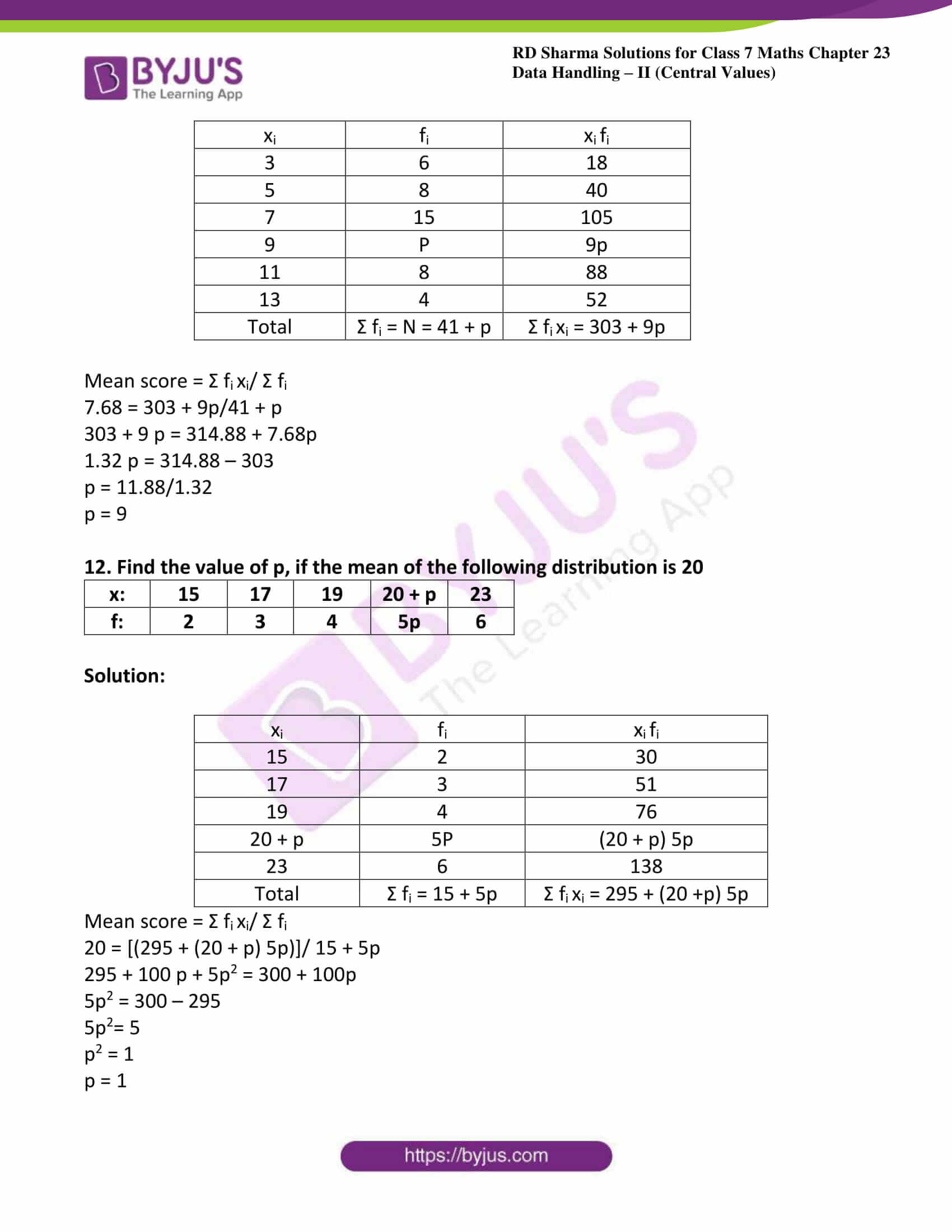### Access answers to Maths RD Sharma Solutions For Class 7 Chapter 23 – Data Handling – II (Central values) Exercise 23.2

1. A die was thrown 20 times and the following scores were recorded:

5, 2, 1, 3, 4, 4, 5, 6, 2, 2, 4, 5, 5, 6, 2, 2, 4, 5, 5, 1

Prepare the frequency table of the scores on the upper face of the die and find the mean score.

Solution:

The frequency table for the given data is as follows:

 x: 1 2 3 4 5 6 f: 2 5 1 4 6 2

To compute arithmetic mean we have to prepare the following table:

 Scores (xi) Frequency (fi) xi fi 1 2 2 2 5 10 3 1 3 4 4 16 5 6 30 6 2 12 Total Σ fi = 20 Σ fi xi

Mean score = Σ fi xi/ Σ fi

= 73/20

= 3.65

2. The daily wages (in Rs) of 15 workers in a factory are given below:

200, 180, 150, 150, 130, 180, 180, 200, 150, 130, 180, 180, 200, 150, 180

Prepare the frequency table and find the mean wage.

Solution:

 Wages (xi) 130 150 180 200 Number of workers (fi) 2 4 6 3

To compute arithmetic mean we have to prepare the following table:

 xi fi xi fi 130 2 260 150 4 600 180 6 1080 200 3 600 Total Σ fi = N = 15 Σ fi xi = 2540

Mean score = Σ fi xi/ Σ fi

= 2540/15

= 169.33

3. The following table shows the weights (in kg) of 15 workers in a factory:

 Weight (in Kg) 60 63 66 72 75 Number of workers 4 5 3 1 2

Calculate the mean weight.

Solution:

Calculation of mean:

 xi fi xi fi 60 4 240 63 5 315 66 3 198 72 1 72 75 2 150 Total Σ fi = N = 15 Σ fi xi = 975

Mean score = Σ fi xi/ Σ fi

= 975/15

= 65 kg

4. The ages (in years) of 50 students of a class in a school are given below:

 Age (in years) 14 15 16 17 18 Number of students 15 14 10 8 3

Find the mean age.

Solution:

Calculation of mean:

 xi fi xi fi 14 15 210 15 14 210 16 10 160 17 8 136 18 3 54 Total Σ fi = N = 50 Σ fi xi = 770

Mean score = Σ fi xi/ Σ fi

= 770/50

= 15.4 years

5. Calculate the mean for the following distribution:

 x: 5 6 7 8 9 f: 4 8 14 11 3

Solution:

 xi fi xi fi 5 4 20 6 8 48 7 14 98 8 11 88 9 3 27 Total Σ fi = N = 40 Σ fi xi = 281

Mean score = Σ fi xi/ Σ fi

= 281/40

= 7.025

6. Find the mean of the following data:

 x: 19 21 23 25 27 29 31 f: 13 15 16 18 16 15 13

Solution:

 xi fi xi fi 19 13 247 21 15 315 23 16 368 25 18 450 27 16 432 29 15 435 31 13 403 Total Σ fi = N = 106 Σ fi xi = 2650

Mean score = Σ fi xi/ Σ fi

= 2650/106

= 25

7. The mean of the following data is 20.6. Find the value of p.

 x: 10 15 p 25 35 f: 3 10 25 7 5

Solution:

 xi fi xi fi 10 3 30 15 10 150 P 25 25p 25 7 175 35 5 175 Total Σ fi = N = 50 Σ fi xi = 530 + 25p

Mean score = Σ fi xi/ Σ fi

20.6 = 530 + 25p/50

530 + 25 p = 20.6 x 50

25 p = 1030 – 530

p = 500/25

p = 20

8. If the mean of the following data is 15, find p.

 x: 5 10 15 20 25 f: 6 p 6 10 5

Solution:

 xi fi xi fi 5 6 30 10 P 10p 15 6 90 20 10 200 25 5 125 Total Σ fi = 27 + p Σ fi xi = 445 + 10p

Mean score = Σ fi xi/ Σ fi

15 = 445 + 10p/27 + p

445 + 10 p = 405 + 15p

5 p = 445 – 405

p = 40/5

p = 8

9. Find the value of p for the following distribution whose mean is 16.6

 x: 8 12 15 p 20 25 30 f: 12 16 20 24 16 8 4

Solution:

 xi fi xi fi 8 12 96 12 16 192 15 20 300 P 24 24p 20 16 320 25 8 200 30 4 120 Total Σ fi = N = 100 Σ fi xi = 1228 + 24p

Mean score = Σ fi xi/ Σ fi

16.6 = 1228 + 24p/100

1228 + 24 p = 16.6 x 100

24 p = 1660 – 1228

p = 432/24

p = 18

10. Find the missing value of p for the following distribution whose mean is 12.58

 x: 5 8 10 12 p 20 25 f: 2 5 8 22 7 4 2

Solution:

 xi fi xi fi 5 2 10 8 5 40 10 8 80 12 22 264 P 7 7p 20 4 80 25 2 50 Total Σ fi = N = 50 Σ fi xi = 524 + 7p

Mean score = Σ fi xi/ Σ fi

12.58 = 524 + 7p/50

524 + 7 p = 12.58 x 50

7 p = 629 – 524

p = 105/7

p = 15

11. Find the missing frequency (p) for the following distribution whose mean is 7.68

 x: 3 5 7 9 11 13 f: 6 8 15 p 8 4

Solution:

 xi fi xi fi 3 6 18 5 8 40 7 15 105 9 P 9p 11 8 88 13 4 52 Total Σ fi = N = 41 + p Σ fi xi = 303 + 9p

Mean score = Σ fi xi/ Σ fi

7.68 = 303 + 9p/41 + p

303 + 9 p = 314.88 + 7.68p

1.32 p = 314.88 – 303

p = 11.88/1.32

p = 9

12. Find the value of p, if the mean of the following distribution is 20

 x: 15 17 19 20 + p 23 f: 2 3 4 5p 6

Solution:

 xi fi xi fi 15 2 30 17 3 51 19 4 76 20 + p 5P (20 + p) 5p 23 6 138 Total Σ fi = 15 + 5p Σ fi xi = 295 + (20 +p) 5p

Mean score = Σ fi xi/ Σ fi

20 = [(295 + (20 + p) 5p)]/ 15 + 5p

295 + 100 p + 5p2 = 300 + 100p

5p2 = 300 – 295

5p2= 5

p2 = 1

p = 1